# Surface of the second order

A set of points in the three-dimensional real or complex space whose coordinates in a Cartesian coordinate system satisfy an algebraic equation of degree two:(*)Equation (*) need not define a real geometric image, and in such cases one says that (*) defines an imaginary second-order surface. Depending on the coefficients in the general equation (*), one may transform it by parallel translation and rotation in the coordinate system to one of the 17 canonical forms given below, each of which corresponds to a certain class of surfaces. Precisely, the non-singular irreducible surfaces: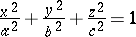(an ellipsoid),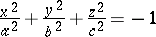(an imaginary ellipsoid),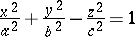(a one-sheet hyperboloid),(a two-sheet hyperboloid),,(an elliptic paraboloid),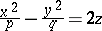,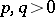(a hyperbolic paraboloid);

the singular irreducible surfaces: the cylindrical surfaces (cf. Cylindrical surface (cylinder)) —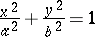(an elliptic cylinder),(an imaginary elliptic cylinder),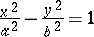(a hyperbolic cylinder),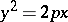(a parabolic cylinder);

the conical surfaces (cf. Conical surface) —(a conical surface),(an imaginary conical surface);

the singular reducible surfaces: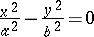(a pair of intersecting planes),(a pair of imaginary intersecting planes),(a pair of parallel planes),(a pair of imaginary parallel planes),(a pair of coincident planes).

A second-order surface having a unique centre of symmetry (the centre of the surface) is called a central surface. The coordinates of the centre are defined as the solution to the system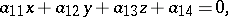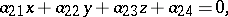A second-order surface without a centre of symmetry or with an indeterminate centre is called a non-central surface.

One can examine second-order surfaces without reducing the general equation to a canonical form by the joint consideration of the so-called basic invariants of second-order surfaces. These are expressions made up from the coefficients of (*) whose values do not alter under parallel translation and rotation of the coordinate system: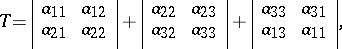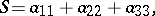together with the semi-invariantsand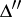, which are invariant under rotation of the coordinate system:whereis the algebraic complement of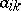in, and'

<tbody> </tbody>
 Non-singular surfaces Singular surfaces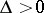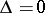Central surfaces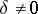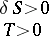Imaginary ellipsoid Ellipsoid Imaginary coneOne-sheet hyperboloid Two-sheet hyperboloid Real cone Non-central surfacesHyperbolic paraboloid Elliptic paraboloid Cylindrical and reducible surfaces (cf. Table 2)

The invariants, in general, define a second-order surface up to a motion in Euclidean space; if corresponding invariants for two surfaces are equal, these surfaces may be brought to coincide by a motion. In other words, these surfaces are equivalent in relation to the group of spatial motions (are metrically equivalent).'

<tbody> </tbody>
 Cylindrical surfaces Reducible surfaces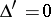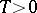Elliptic cylinder Imaginary () Real () A pair of imaginary intersecting planesHyperbolic cylinder A pair of intersecting planes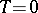Parabolic cylinder A pair of imaginary parallel planes (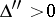) A pair of parallel planes (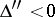) A pair of coincident planes ()

There is a classification of second-order surfaces from the point of view of other transformation groups. For example, with respect to the group of affine transformations, equivalence applies for any two surfaces defined by equations of the same canonical form, e.g. two similar second-order surfaces are equivalent.

The relationships between the various affine classes of second-order surfaces enable one to establish a classification from the point of view of projective geometry. Here one takes as equivalent those surfaces that can be mapped onto another by means of projective transformations. For example, ellipsoids, elliptic paraboloids and two-sheet hyperboloids are real oval surfaces from the point of view of projective geometry. Their projective equivalence manifests itself in that there is a certain system of projective coordinates in which the equations for these surfaces are identical in form: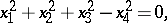i.e. the corresponding quadratic forms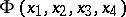have identical rank

and identical signature . Their affine difference appears in the type of the line of intersection with the improper plane: an ellipsoid intersects it in an imaginary oval, a hyperboloid in a real oval and an elliptic paraboloid in a pair of imaginary intersecting straight lines. In all, there are eight projective equivalence classes for second-order surfaces: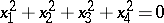(an imaginary oval surface),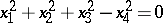(a real oval surface),(an annular surface),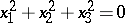(an imaginary conical surface),(a real conical surface),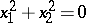(a pair of imaginary planes),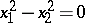(a pair of real planes),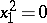(a pair of coincident planes).

For references see Second-order curve.

Similar classifications are possible over other fields, such as, finite fields and-adic fields.